Anúncio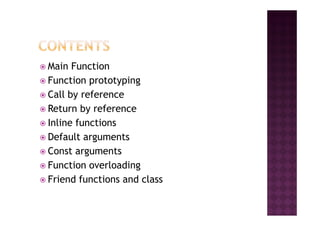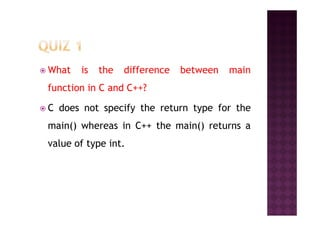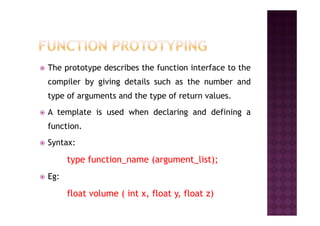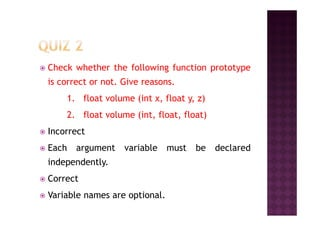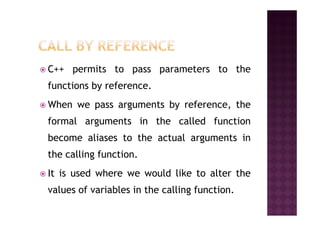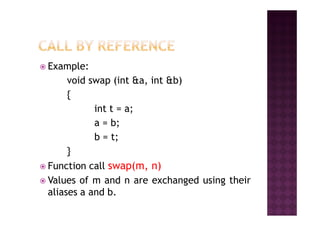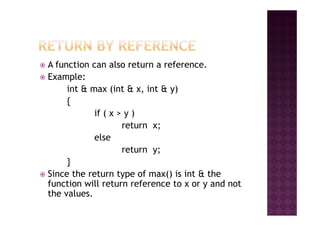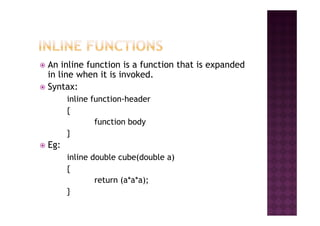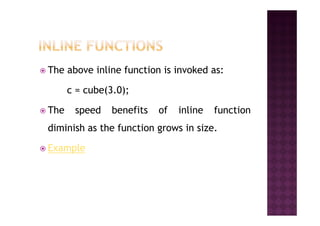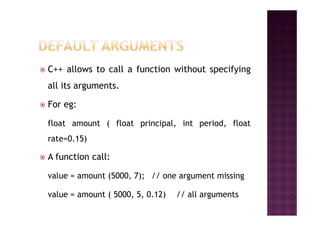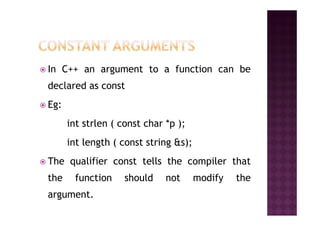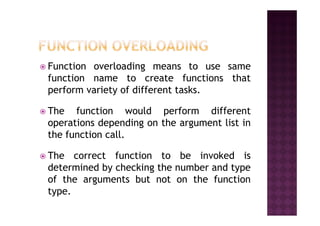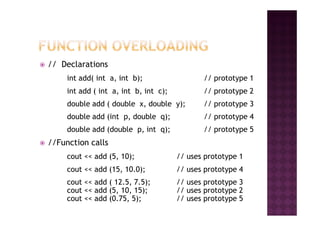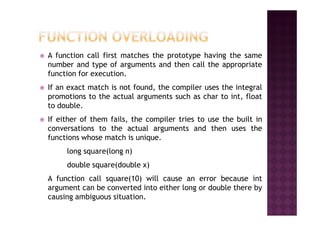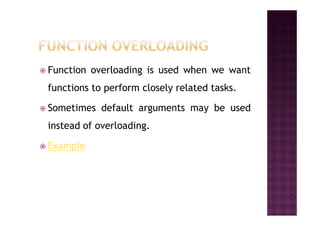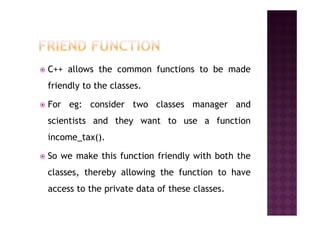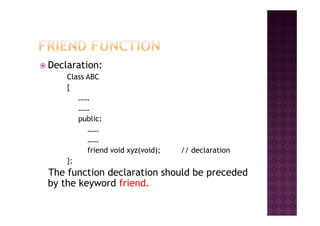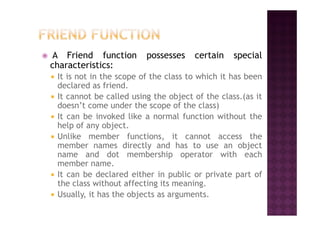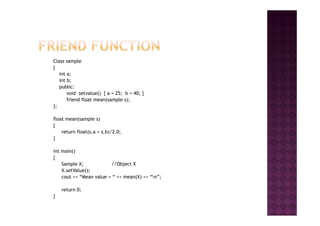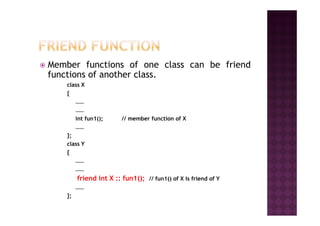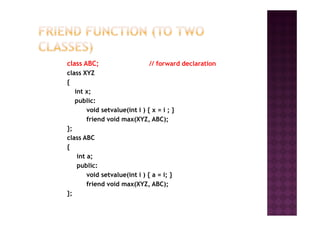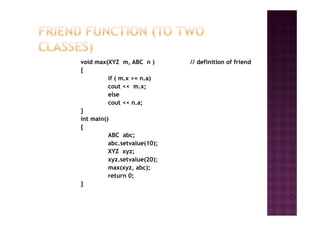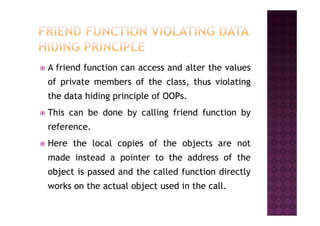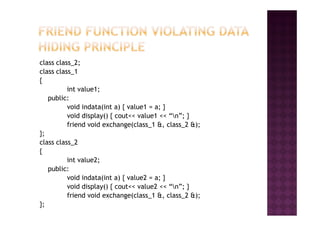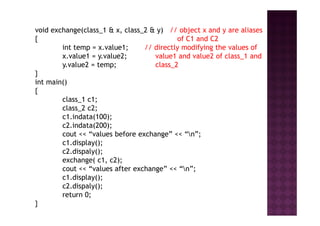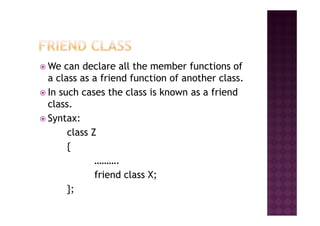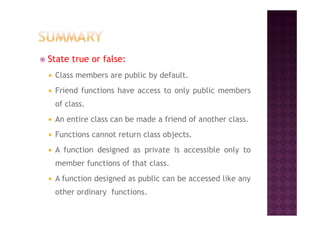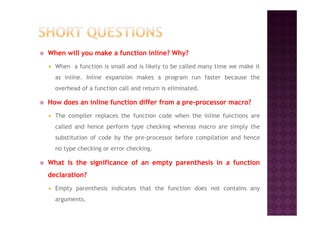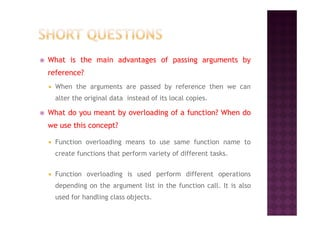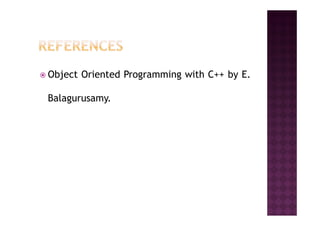1 de 32
Anúncio

### Functions in C++

1. Mrs. Pranali P. Chaudhari
2. Main Function Function prototyping Call by reference Return by referenceReturn by reference Inline functions Default arguments Const arguments Function overloading Friend functions and class
3. What is the difference between main function in C and C++? C does not specify the return type for theC does not specify the return type for the main() whereas in C++ the main() returns a value of type int.
4. The prototype describes the function interface to the compiler by giving details such as the number and type of arguments and the type of return values. A template is used when declaring and defining aA template is used when declaring and defining a function. Syntax: type function_name (argument_list); Eg: float volume ( int x, float y, float z)
5. Check whether the following function prototype is correct or not. Give reasons. 1. float volume (int x, float y, z) 2. float volume (int, float, float)2. float volume (int, float, float) Incorrect Each argument variable must be declared independently. Correct Variable names are optional.
6. C++ permits to pass parameters to the functions by reference. When we pass arguments by reference, the formal arguments in the called function become aliases to the actual arguments in the calling function. It is used where we would like to alter the values of variables in the calling function.
7. Example: void swap (int &a, int &b) { int t = a;int t = a; a = b; b = t; } Function call swap(m, n) Values of m and n are exchanged using their aliases a and b.
8. A function can also return a reference. Example: int & max (int & x, int & y) { if ( x > y )if ( x > y ) return x; else return y; } Since the return type of max() is int & the function will return reference to x or y and not the values.
9. An inline function is a function that is expanded in line when it is invoked. Syntax: inline function-header {{ function body } Eg: inline double cube(double a) { return (a*a*a); }
10. The above inline function is invoked as: c = cube(3.0); The speed benefits of inline functionThe speed benefits of inline function diminish as the function grows in size. Example
11. C++ allows to call a function without specifying all its arguments. For eg: float amount ( float principal, int period, float rate=0.15) A function call: value = amount (5000, 7); // one argument missing value = amount ( 5000, 5, 0.12) // all arguments
12. In C++ an argument to a function can be declared as const Eg: int strlen ( const char *p ); int length ( const string &s); The qualifier const tells the compiler that the function should not modify the argument.
13. Function overloading means to use same function name to create functions that perform variety of different tasks. The function would perform differentThe function would perform different operations depending on the argument list in the function call. The correct function to be invoked is determined by checking the number and type of the arguments but not on the function type.
15. A function call first matches the prototype having the same number and type of arguments and then call the appropriate function for execution. If an exact match is not found, the compiler uses the integral promotions to the actual arguments such as char to int, float to double. If either of them fails, the compiler tries to use the built in conversations to the actual arguments and then uses the functions whose match is unique. long square(long n) double square(double x) A function call square(10) will cause an error because int argument can be converted into either long or double there by causing ambiguous situation.
17. C++ allows the common functions to be made friendly to the classes. For eg: consider two classes manager and scientists and they want to use a function income_tax(). So we make this function friendly with both the classes, thereby allowing the function to have access to the private data of these classes.
18. Declaration: Class ABC { …… …… public: …… …… friend void xyz(void); // declaration }; The function declaration should be preceded by the keyword friend.
19. A Friend function possesses certain special characteristics: It is not in the scope of the class to which it has been declared as friend. It cannot be called using the object of the class.(as it doesn’t come under the scope of the class) It can be invoked like a normal function without theIt can be invoked like a normal function without the help of any object. Unlike member functions, it cannot access the member names directly and has to use an object name and dot membership operator with each member name. It can be declared either in public or private part of the class without affecting its meaning. Usually, it has the objects as arguments.
20. Class sample { int a; int b; public: void setvalue() { a = 25; b = 40; } friend float mean(sample s); }; float mean(sample s) { return float(s.a + s.b)/2.0; } int main() { Sample X; //Object X X.setValue(); cout << “Mean value = “ << mean(X) << “n”; return 0; }
21. Member functions of one class can be friend functions of another class. class X { …… ………… int fun1(); // member function of X …… }; class Y { …… …… friend int X :: fun1(); // fun1() of X is friend of Y …… };
22. class ABC; // forward declaration class XYZ { int x; public: void setvalue(int i ) { x = i ; }void setvalue(int i ) { x = i ; } friend void max(XYZ, ABC); }; class ABC { int a; public: void setvalue(int i ) { a = i; } friend void max(XYZ, ABC); };
23. void max(XYZ m, ABC n ) // definition of friend { if ( m.x >= n.a) cout << m.x; else cout << n.a; }} int main() { ABC abc; abc.setvalue(10); XYZ xyz; xyz.setvalue(20); max(xyz, abc); return 0; }
24. A friend function can access and alter the values of private members of the class, thus violating the data hiding principle of OOPs. This can be done by calling friend function byThis can be done by calling friend function by reference. Here the local copies of the objects are not made instead a pointer to the address of the object is passed and the called function directly works on the actual object used in the call.
25. class class_2; class class_1 { int value1; public: void indata(int a) { value1 = a; } void display() { cout<< value1 << “n”; }void display() { cout<< value1 << “n”; } friend void exchange(class_1 &, class_2 &); }; class class_2 { int value2; public: void indata(int a) { value2 = a; } void display() { cout<< value2 << “n”; } friend void exchange(class_1 &, class_2 &); };
26. void exchange(class_1 & x, class_2 & y) // object x and y are aliases { of C1 and C2 int temp = x.value1; // directly modifying the values of x.value1 = y.value2; value1 and value2 of class_1 and y.value2 = temp; class_2 } int main() { class_1 c1; class_2 c2; c1.indata(100);c1.indata(100); c2.indata(200); cout << “values before exchange” << “n”; c1.display(); c2.dispaly(); exchange( c1, c2); cout << “values after exchange” << “n”; c1.display(); c2.dispaly(); return 0; }
27. We can declare all the member functions of a class as a friend function of another class. In such cases the class is known as a friend class. Syntax:Syntax: class Z { ………. friend class X; };
28. State true or false: Class members are public by default. Friend functions have access to only public members of class. An entire class can be made a friend of another class. Functions cannot return class objects. A function designed as private is accessible only to member functions of that class. A function designed as public can be accessed like any other ordinary functions.
29. When will you make a function inline? Why? When a function is small and is likely to be called many time we make it as inline. Inline expansion makes a program run faster because the overhead of a function call and return is eliminated. How does an inline function differ from a pre-processor macro? The compiler replaces the function code when the inline functions areThe compiler replaces the function code when the inline functions are called and hence perform type checking whereas macro are simply the substitution of code by the pre-processor before compilation and hence no type checking or error checking. What is the significance of an empty parenthesis in a function declaration? Empty parenthesis indicates that the function does not contains any arguments.